Test: Effect of Source Impedence on the Performance of Converters

# Test: Effect of Source Impedence on the Performance of Converters - Electrical Engineering (EE)

Test Description

## 10 Questions MCQ Test Power Electronics - Test: Effect of Source Impedence on the Performance of Converters

Test: Effect of Source Impedence on the Performance of Converters for Electrical Engineering (EE) 2023 is part of Power Electronics preparation. The Test: Effect of Source Impedence on the Performance of Converters questions and answers have been prepared according to the Electrical Engineering (EE) exam syllabus.The Test: Effect of Source Impedence on the Performance of Converters MCQs are made for Electrical Engineering (EE) 2023 Exam. Find important definitions, questions, notes, meanings, examples, exercises, MCQs and online tests for Test: Effect of Source Impedence on the Performance of Converters below.
Solutions of Test: Effect of Source Impedence on the Performance of Converters questions in English are available as part of our Power Electronics for Electrical Engineering (EE) & Test: Effect of Source Impedence on the Performance of Converters solutions in Hindi for Power Electronics course. Download more important topics, notes, lectures and mock test series for Electrical Engineering (EE) Exam by signing up for free. Attempt Test: Effect of Source Impedence on the Performance of Converters | 10 questions in 30 minutes | Mock test for Electrical Engineering (EE) preparation | Free important questions MCQ to study Power Electronics for Electrical Engineering (EE) Exam | Download free PDF with solutions
 1 Crore+ students have signed up on EduRev. Have you?
Test: Effect of Source Impedence on the Performance of Converters - Question 1

### Consider following statements : The overlap angle of single phase fully controlled bridge converter would increase an increasing Supply voltage Supply frequency Load current Source inductance Of these statements

Detailed Solution for Test: Effect of Source Impedence on the Performance of Converters - Question 1

Effect of source inductance on 1ϕ full controlled rectifier:
For 1ϕ full wave converter, the average reduction in dc output voltage due to source inductance is: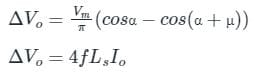where, Δ Vo =  Average reduction in dc output voltage
Vm = Maximum value of source voltage
α = Firing angle
μ = Overlap angle
LS = Source inductance

• If f ↑ , Ls ↑, Io ↑, without changing the Vm and α, then μ also increases.
• If Vm ↑ , without changing f, Ls, Io, and α, then μ decreases.

Hence, option 4 is correct.

Test: Effect of Source Impedence on the Performance of Converters - Question 2

### A half-wave, controlled rectifier with a purely resistive load has delay α = π/3, Calculate form factor.

Detailed Solution for Test: Effect of Source Impedence on the Performance of Converters - Question 2

Concept-
If a gate signal is applied to the SCR at ωt = α, where α is the delay (firing or triggering) angle. The average (dc) voltage across the load resistor is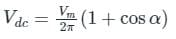The rms voltage Across the Resistor is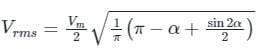FORM factor for Rectifier is given by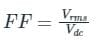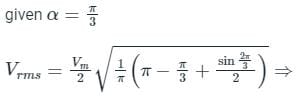Given:
α = π/3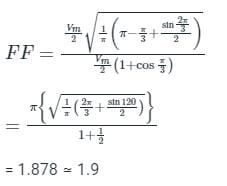Test: Effect of Source Impedence on the Performance of Converters - Question 3

### What is the maximum output voltage of 3-phase full bridge rectifier supplied with a line voltage of 420 V?

Detailed Solution for Test: Effect of Source Impedence on the Performance of Converters - Question 3

Concept:
The average output voltage of a three-phase bridge rectifier is given by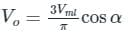Maximum possible output voltage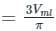Calculation:
Given that, line voltage (VL) = 420 V
Maximum line voltage = 420 × √2 V
Maximum possible output voltage
Maximum possible output voltage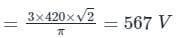*Answer can only contain numeric values
Test: Effect of Source Impedence on the Performance of Converters - Question 4

A single-phase, full-bridge, fully controlled thyristor rectifier feeds a load comprising a 10 Ω resistance in series with a very large inductance. The rectifier is fed from an ideal 230 V, 50 Hz sinusoidal source through cables which have negligible internal resistance and a total inductance of 2.28 mH. If the thyristors are triggered at an angle α = 45°, the commutation overlap angle in degree (rounded off to 2 decimal places) is _______

Detailed Solution for Test: Effect of Source Impedence on the Performance of Converters - Question 4

Concept:
In a single-phase full wave rectifier with source inductance is given by,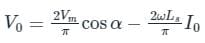Average output current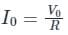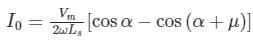Where, Vm is the maximum value of supply voltage
α is the firing angle
μ is the overlapping angle
Ls is the source inductance

Calculation:
Given that, supply voltage (Vrms) = 230 V
Firing angle (α) = 45°
Source inductance (Ls) = 2.28 mH
Load resistance (R) = 10 Ω
Frequency (f) = 50 Hz
The average output voltage is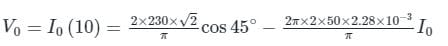⇒ I0 = 14.003 A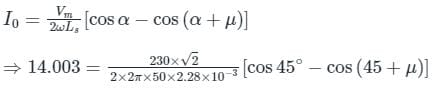⇒ μ = 4.8°

*Answer can only contain numeric values
Test: Effect of Source Impedence on the Performance of Converters - Question 5

The figure below shows an uncontrolled diode bridge rectifier supplied from a 220 V, 50 Hz, 1-phase ac source. The load draws a constant current I0 = 14 A. The conduction angle of the diode D1 in degrees (rounded off to two decimal places) is ________.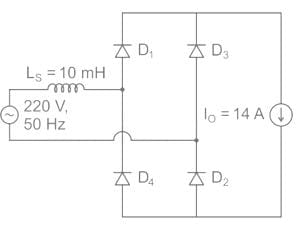Detailed Solution for Test: Effect of Source Impedence on the Performance of Converters - Question 5

Concept:
The small inductance connected with supply work as a source inductance Due to this inductance, there will be overlapping takes place between two diodes. As a result, this will cause an overlap angle 'μ'.
During overlap period, both diode D1 & D2 will conduct.

Calculation:
The output voltage (V0) & current waveform is shown below,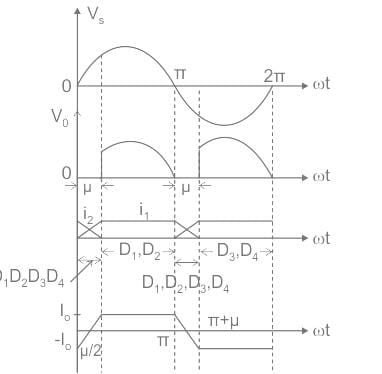Output voltage in terms of load current is given by,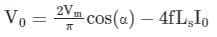For diode α = 0°,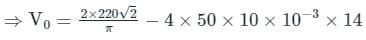⇒ V0 = 170.06 V
In terms of overlap angle,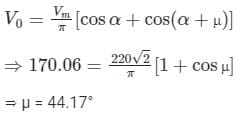Since, for an overlap angle of μ, all four diodes conduct & each diodes conducts for (180 + μ) degree.
So,
conduction angle (γ) = 180 + μ = 180 + 44.17
γ = 224.17°

Test: Effect of Source Impedence on the Performance of Converters - Question 6

A three-phase three pulse converter, fed from three-phase 400 V, 50Hz supply, has a load R = 2 Ω, E = 200 V, and large inductance so that load current is constant at 20A. If the source has an inductance of 2 mH, then the value of overlap angle for inverter operation is

Detailed Solution for Test: Effect of Source Impedence on the Performance of Converters - Question 6

Concept:
Effect of source inductance: The presence of source inductance affects the rectifier output voltage
V0 = Vd0 cos α – ΔVd0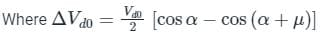μ is the overlap angle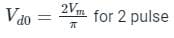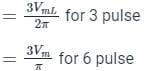Also, ΔVd0 = f Ls I0 (1 pulse)
= 4 f Ls I0 (2 pulse)
= 3 f Ls I0 (3 pulse)
= 6 f Ls I0 (6 pulse)

Calculation:
V0 = -E0 + I0 R
Vd0 cos α – 3 f Ls I0 = -200 + (20)(2)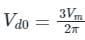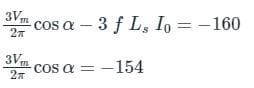On solving we get cos α = - 0.57
⇒ α = 124.76°
For three pulse converters: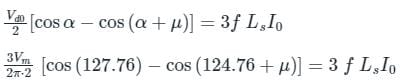On solving the above equation for overlap angle (μ) we get
cos (α + μ) = -0.6145
μ = 3.15°

Test: Effect of Source Impedence on the Performance of Converters - Question 7

For the 3ϕ full converter having resistive load, the ripple frequency f ripple is __________.

Detailed Solution for Test: Effect of Source Impedence on the Performance of Converters - Question 7

Concept:
Ripple Frequency: Ripple frequency is the output frequency of a converter which is some integral multiple of the supply frequency.
fripple= n × fs
fripple= Ripple frequency
fs = Supply frequency
n = No. of pulses in the time period

Calculation:
The 3ϕ full converter has 6 no. of pulses in the time period of the output waveform.
fs = 50 Hz
fripple = 6 × 50
fripple = 300 Hz

Test: Effect of Source Impedence on the Performance of Converters - Question 8

Directions: The question consists of two statements, one labeled as ‘Statement (I)’ and the other labeled as ‘Statement (II)’. You are to examine these two statements carefully and select the answers to these items using the codes given below:
Statement (I): Inductive voltage regulation of single-phase full-wave rectifier increases with increasing supply frequency of the converter.
Statement (II): The overlap angle of a single-phase full-wave rectifier increases with the increase in the supply frequency of the converter.

Detailed Solution for Test: Effect of Source Impedence on the Performance of Converters - Question 8

Concept:
Source inductance effect of single-phase full-wave rectifier:
By considering the source inductance effect the average output voltage of a single-phase full-wave converter is given by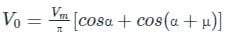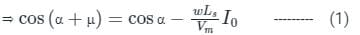Where,
α = firing angle, ω = 2πf = angular frequency of supply
μ = overlap angle, I0 = load current
Inductive voltage regulation of single-phase full-wave rectifier is given by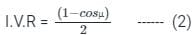Application:
From equation (1), we can observe that
As frequency (ω) of supply increases the cos (α + μ) term decreases. Hence the value of the overlap angle inceases.
Now from equation (2),
As value of overalp angle increases cosμ decreses. Hence the inductive volatge regulation increses.

Test: Effect of Source Impedence on the Performance of Converters - Question 9

A single phase full converter feeds power to RLE load with R = 8Ω, L = 8 mH and E = 46.42 V, the ac source voltage is 230 V, 50 Hz, for continuous conduction. Find the average value of load current for a firing angle delay of 45°

Detailed Solution for Test: Effect of Source Impedence on the Performance of Converters - Question 9

The average output voltage of a 1-ϕ full converter with RLE loads for continuous conduction.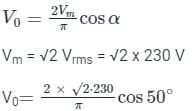V0 = 146.42 V
V0 = E + I0R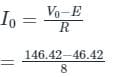I0 = 12.50 A

Test: Effect of Source Impedence on the Performance of Converters - Question 10

The frequency of ripple in the output voltage of a three phase controlled bridge rectifier depends on

Detailed Solution for Test: Effect of Source Impedence on the Performance of Converters - Question 10

The frequency of ripple in the output voltage of a three-phase controlled bridge rectifier depends on the supply frequency. The output frequency is
f0 = n fS
Where n is the number of pulses in the output of the converter and fs is the supply frequency
Note: Ripple factor depends on the firing angle, load inductance, and resistance.

## Power Electronics

5 videos|39 docs|63 tests
Information about Test: Effect of Source Impedence on the Performance of Converters Page
In this test you can find the Exam questions for Test: Effect of Source Impedence on the Performance of Converters solved & explained in the simplest way possible. Besides giving Questions and answers for Test: Effect of Source Impedence on the Performance of Converters, EduRev gives you an ample number of Online tests for practice

## Power Electronics

5 videos|39 docs|63 tests(Scan QR code)